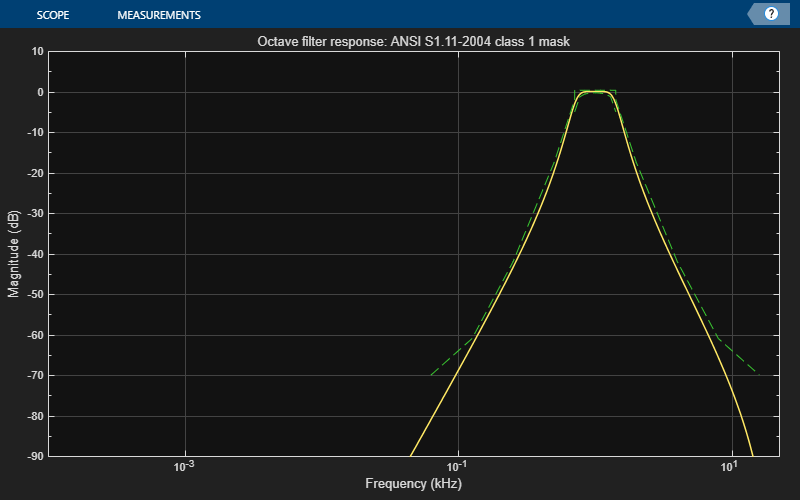# visualize

Visualize and validate filter response

## Syntax

``visualize(octFilt)``
``visualize(octFilt,N)``
``visualize(___,mType)``

## Description

example

````visualize(octFilt)` plots the magnitude response of the octave-band filter, `octFilt`. The plot is updated automatically when properties of the object change.```

example

````visualize(octFilt,N)` uses an `N`-point FFT to calculate the magnitude response.```

example

````visualize(___,mType)` creates a mask based on the class of filter specified by `mType`, using either of the previous syntaxes. Specify `mType` as ```'class 0'```, `'class 1'`, or `'class 2'`. The mask attenuation limits are defined in the ANSI S1.11-2004 standard. The mask center frequency is the ANSI standard center frequency, with band edge frequencies on either side of the `CenterFrequency` set in `octFilt`. If the mask is green, the design is compliant with the ANSI S1.11-2004 standard.If the mask is red, the design breaks compliance. ```

## Examples

collapse all

Create an object of the `octaveFilter` System object™ and then plot the magnitude response of the filter.

```octFilt = octaveFilter; visualize(octFilt)```Create an object of the `octaveFilter` System object™. Plot a 5096-point frequency representation.

```octFilt = octaveFilter; visualize(octFilt,5096)```Create an object of the `octaveFilter` System object™. Visualize the class 1 compliance of the filter design.

```octFilt = octaveFilter; visualize(octFilt,'class 1')```## Input Arguments

collapse all

Object of the `octaveFilter` System object™.

Number of DFT bins in frequency-domain representation, specified as a positive scalar. The default is `2048`.

Data Types: `single` | `double`

Type of mask, specified as `'class 0'`, ```'class 1```, or `'class 2'`.

The mask attenuation limits are defined in the ANSI S1.11-2004 standard. The mask center frequency is the ANSI standard center frequency, with band edge frequencies on either side of the `CenterFrequency` set in `octFilt`.

• If the mask is green, the design is compliant with the ANSI S1.11-2004 standard.

• If the mask is red, the design breaks compliance.

Data Types: `char`